# Division Flashcards

Division Flashcards
1/43 (missed) 0 0
Create Your Account To Continue Studying

As a member, you'll also get unlimited access to over 79,000 lessons in math, English, science, history, and more. Plus, get practice tests, quizzes, and personalized coaching to help you succeed.

Try it risk-free for 30 days. Cancel anytime
Solve for the quotient.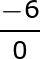The quotient is undefined.
Got it
Solve for the quotient.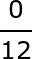The quotient is 0.
Got it
If a fraction is undefined, what must the denominator be?
The denominator must be 0.
Got it
If a fraction is equal to zero, what must the numerator be?
The numerator must be 0.
Got it
Solve for the quotient.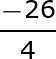The quotient is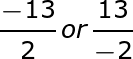Got it
Solve for the quotient.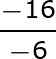The quotient is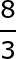Got it
Solve for the quotient.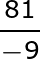The quotient is -9.
Got it
Solve for the quotient.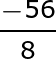The quotient is -7.
Got it
When you divide a positive number and a negative number, will your quotient be positive or negative?
The quotient will be negative.
Got it
Solve for the quotient.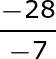The quotient is 4 or +4.
Got it
When you divide two negative numbers, will your quotient be positive or negative?
The quotient will be positive.
Got it
When you divide two positive numbers, will your quotient be positive or negative?
The quotient will be positive.
Got it
Solve for the quotient.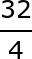The quotient is 8 or +8.
Got it
In the example, which number is the denominator?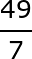The denominator is 7.
Got it
In the example, which number is the numerator?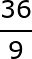The numerator is 36.
Got it
What is a denominator?
A denominator is the digit(s) on the bottom of a fraction.
Got it
What is a numerator?
A numerator is the digit(s) on the top of a fraction.
Got it
What digit is the quotient in the following example?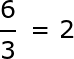The quotient is 2.
Got it
What is a quotient?
A quotient is the answer you get when you divide one number by another.
Got it

True or False?

Every number except zero is either negative or positive.

True
Got it

or choose a specific lesson: See all lessons in this chapter
43 cards in set

## Flashcard Content Overview

Math, especially division, is everywhere around us. Let's say you were planning a birthday party and you wanted to serve pizza to your friends. You would need to use division to decide how many slices you would need, and ultimately, how many pizzas to order.

Let's look at another example. You bought bags of gummy worms from the candy store and you want to share with your family. You would need to use division to figure out how many gummy worms each family member would get. Whether you realize it or not, you use division every day. These flashcards will give you the chance to reinforce your division skills through practice.

Front
Back

True or False?

Every number except zero is either negative or positive.

True
What is a quotient?
A quotient is the answer you get when you divide one number by another.
What digit is the quotient in the following example?The quotient is 2.
What is a numerator?
A numerator is the digit(s) on the top of a fraction.
What is a denominator?
A denominator is the digit(s) on the bottom of a fraction.
In the example, which number is the numerator?The numerator is 36.
In the example, which number is the denominator?The denominator is 7.
Solve for the quotient.The quotient is 8 or +8.
When you divide two positive numbers, will your quotient be positive or negative?
The quotient will be positive.
When you divide two negative numbers, will your quotient be positive or negative?
The quotient will be positive.
Solve for the quotient.The quotient is 4 or +4.
When you divide a positive number and a negative number, will your quotient be positive or negative?
The quotient will be negative.
Solve for the quotient.The quotient is -7.
Solve for the quotient.The quotient is -9.
Solve for the quotient.The quotient isSolve for the quotient.The quotient isIf a fraction is equal to zero, what must the numerator be?
The numerator must be 0.
If a fraction is undefined, what must the denominator be?
The denominator must be 0.
Solve for the quotient.The quotient is 0.
Solve for the quotient.The quotient is undefined.

True or false:

Undefined is the same as zero.

False.
Solve for the quotient.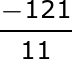The quotient is -11.
Solve for the quotient.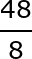The quotient is 6 or +6.
Solve for the quotient.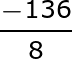The quotient is 17 or +17.
Solve for the quotient.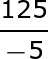The quotient is -25.
Paws 'n Tails Pet Store has 24 tropical fish. They keep 3 fish in each tank. How many fish tanks are there?
There are 8 fish tanks.
Alexis has 28 cactus plants. She keeps her cactus plants in even rows of 4. How many cactus plants are in each row?
There are 7 cactus plants in each row.
Solve for the quotient.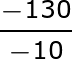The quotient is 13 or +13.
Solve for the quotient.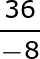The quotient is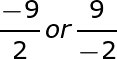Solve for the quotient.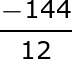The quotient is -12.
Solve for the quotient.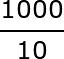The quotient is 100 or +100.
Solve for the quotient.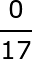The quotient is 0.
Solve for the quotient.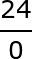The quotient is undefined.
Solve for the quotient.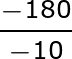The quotient is 18 or +18.
Solve for the quotient.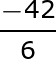The quotient is -7.
Solve for the quotient.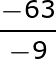The quotient is 7 or +7.
Solve for the quotient.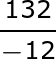The quotient is -11.
Solve for the quotient.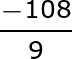The quotient is -12.
Solve for the quotient.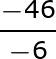The quotient is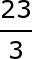Solve for the quotient.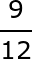The quotient is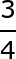Solve for the quotient.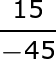The quotient is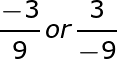Solve for the quotient.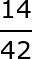The quotient is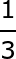Solve for the quotient.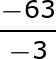The quotient is 21 or +21.

To unlock this flashcard set you must be a Study.com Member.